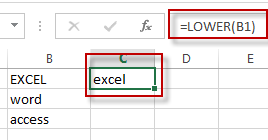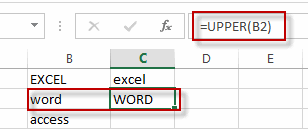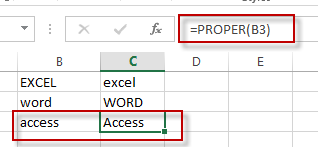# Change Uppercase and Lowercase Text

This post will guide you how to change the case of text to uppercase or lowercase in Excel. How do I change all uppercase text to lower case with an Excel formula.

For example, if you want to change all text string in uppercase into lowercase, you can create a formula based on the Lower function. And if you want to convert all letters in one cell into uppercase, you can use the Upper function as a formula in excel.

If you want to show in Cell C1 a lowercase version of the text in Cell B1, you can type the following formula into the formula box in Cell C1.

=LOWER(B1)If you want to show in Cell C2 an uppercase version of the text in B2, you could type the below formula into the formula box in C2.

=UPPER(B2)If you want to show in Cell C3 a proper version of the text in B3, you could type the below formula into the formula box in C3:

=PROPER(B3)### Related Functions

• Excel UPPER function
The Excel UPPER function converts all characters in text string to uppercase.The UPPER function is a build-in function in Microsoft Excel and it is categorized as a Text Function.The syntax of the UPPER function is as below:= UPPER (text)…
• Excel LOWER function
The Excel LOWER function converts all characters in a text string to lowercase.The syntax of the LOWER function is as below:= LOWER  (text)…
• Excel Proper Function
The Excel PROPER function capitalizes the first character in each word of a text string and set other characters to lowercase. The syntax of the PROPER function is as below:= PROPER  (text)…
Related Posts

Compare effect of (non-annual) compounding periods on growth

This article will talk about how to calculate the trend or effect of future value changes for different compounding periods in a Microsoft Excel spreadsheet or Google Sheets via a formula. What is the period of compound interest? The compounding ...

Coefficient Of Variation in Excel/Google Sheet

This article will talk about how to calculate the coefficient of variation by formula in a Microsoft Excel spreadsheet or Google Sheets.  What is the Coefficient of Variation? The coefficient of variation is the ratio of the standard deviation of ...

VLOOKUP Function & Formula in Excel

In our daily work, we often encounter the situation of finding data based on the original data and conditions provided, such as finding cell phone numbers based on name, finding employee names and positions based on HRID, etc. When we ...

Phone Number Format in Excel

Sometimes when we import phone numbers from external sources, the formatting of the phone numbers is removed. Even if in some situations the phone numbers are not imported from external resource, sometimes the phone numbers in worksheet are not displayed ...

Check Cell If Contains One of Many with Exclusions

In Microsoft Excel Spreadsheet or google sheets, when cells contain multiple strings, how can we accomplish this task if you want to check whether these cells contain more than one given string and exclude other given strings? In this article, ...

Sidebar